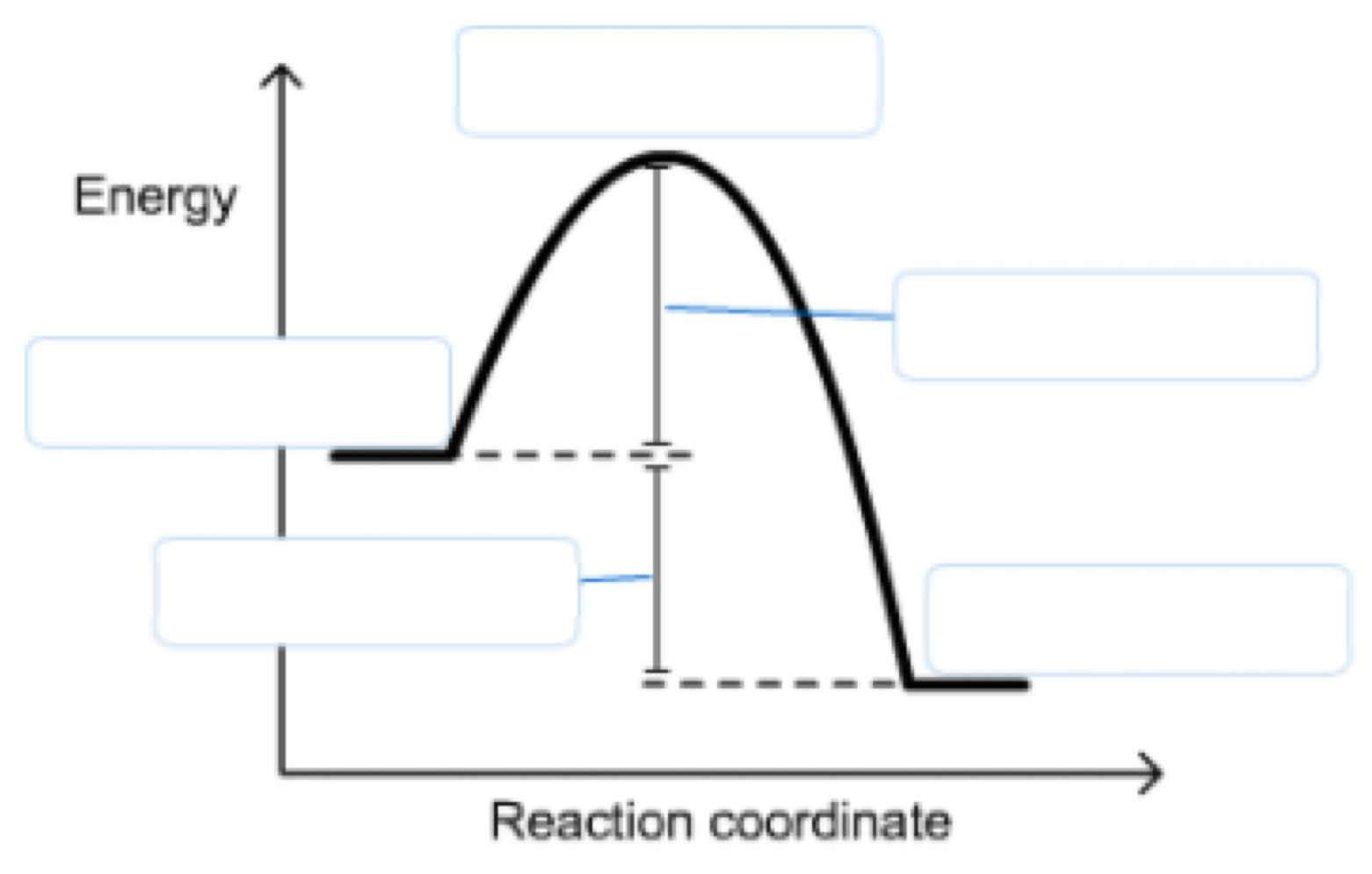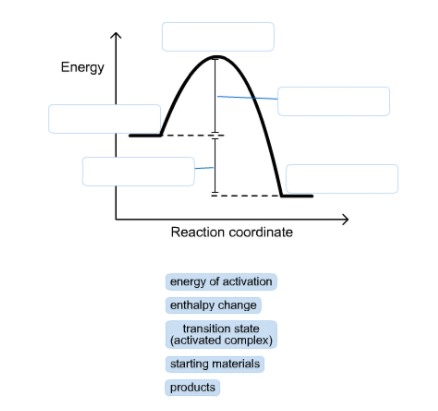# Problem: Label this diagram (energy of activations, transition state, products, starting materials, and enthalpy change)

###### FREE Expert Solution
89% (463 ratings)
###### FREE Expert Solution

We’re being asked to label the given energy diagram.Recall that an energy diagram is usually read from left to right

The components of a one-step energy diagram are:

89% (463 ratings)###### Problem Details

Label this diagram (energy of activations, transition state, products, starting materials, and enthalpy change)What scientific concept do you need to know in order to solve this problem?

Our tutors have indicated that to solve this problem you will need to apply the Energy Diagram concept. If you need more Energy Diagram practice, you can also practice Energy Diagram practice problems.

What is the difficulty of this problem?

Our tutors rated the difficulty ofLabel this diagram (energy of activations, transition state,...as low difficulty.

How long does this problem take to solve?

Our expert Chemistry tutor, Sabrina took 2 minutes and 42 seconds to solve this problem. You can follow their steps in the video explanation above.

What professor is this problem relevant for?

Based on our data, we think this problem is relevant for Professor Jenkins' class at TCC.